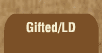Mathematics and Dyslexia

Tips for Learning the Times Tables

Tools for the Times Tables & Workbook

Home > Math > times tables practice > Sixes (small) in order

## The Sixes Times Tables (first set, in order)

If six goes into a number, then 3 *and* 2 will go into it, too. (Can you figure out why?) So, it will be an even number (ends in 2, 4, 6, 8, or 0), *and* the digits will add up to a number that three goes into. So 12 ends in 2, so it's even, and 1 + 2 is 3... which 3 goes into.

1. 6 x 0=
2. 6 x 1=
3. 6 x 2=
4. 6 x 3=
5. 6 x 4=
6. 6 x 5=
7. 5 x 6=
8. 4 x 6=
9. 3 x 6=
10. 2 x 6=
11. 1 x 6=
12. 2 x 6=
13. 6 x 3=
14. 4 x 6=
15. 5 x 6=